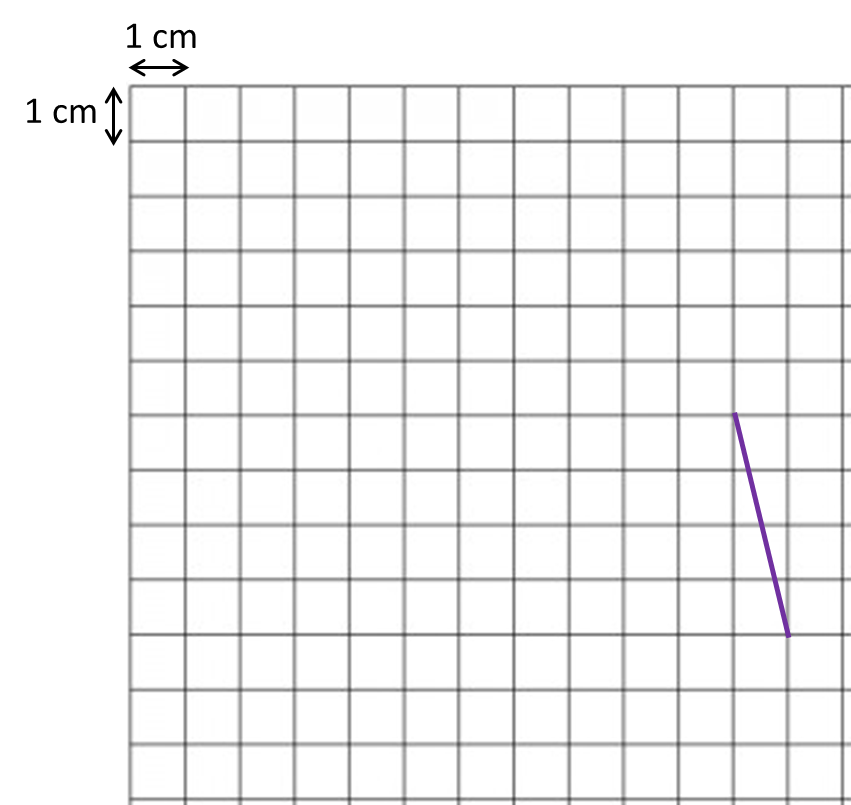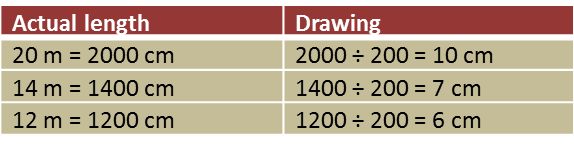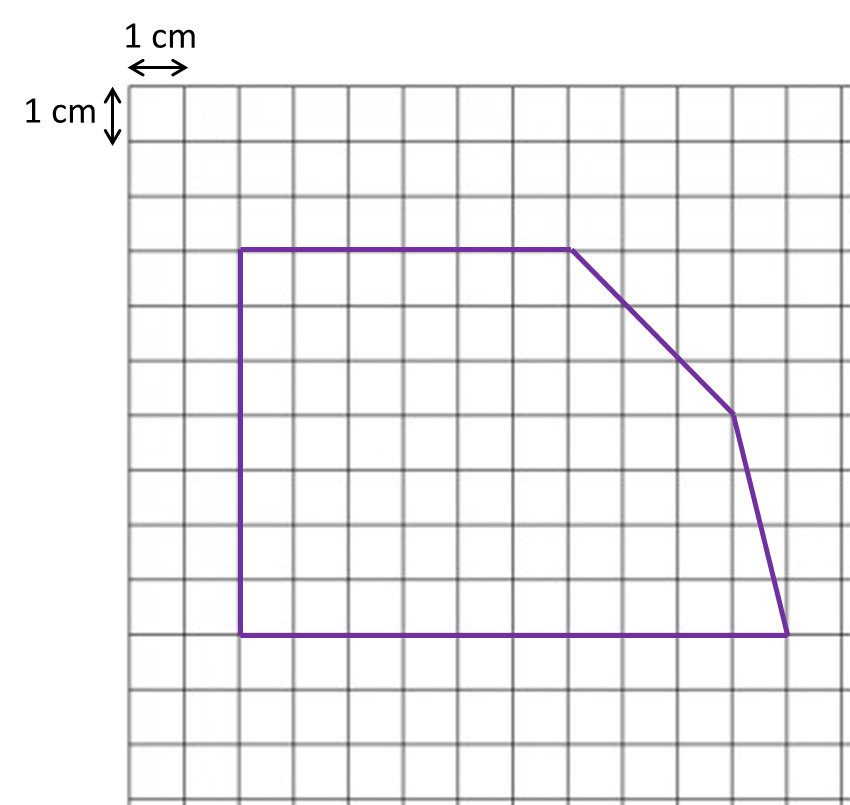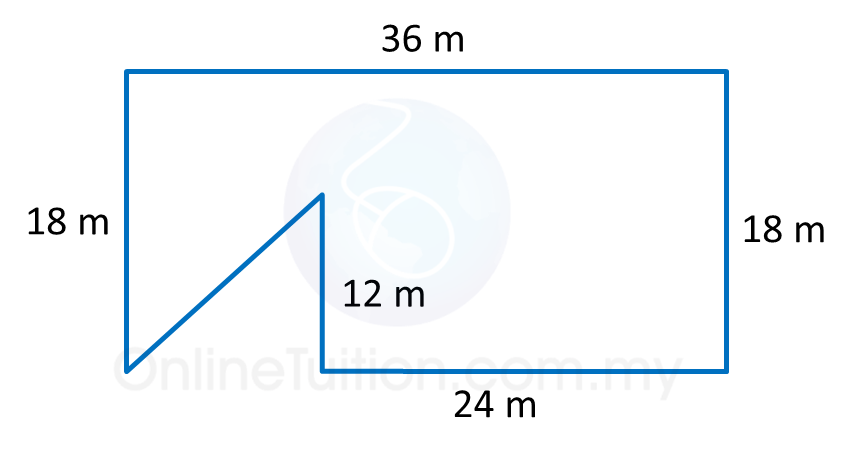# 4.2.3 Scale Drawings, PT3 Focus Practice

Question 6:
Diagram below shows an irregular pentagon.By using the scale 1 : 200, complete the scale drawing in the answer space provided. The grid has equal square with sides of 1 cm.Solution:Question 7:
Diagram below shows a polygon.(a) If the polygon is redrawn using the scale 1 : 300, calculate the length, in cm, the drawing for the side 24 m.
(b) In the answer space, redraw the polygon using the scale 1 : 300. The grid has equal squares with sides of 1 cm.